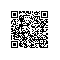# PHP字符串offset取值特性

<?php
$test = "Hello World"; echo$test;    //输出H
echo "<br/>";
echo $test; //输出e ?> Tips：$test 方括号中的数字超出范围将会产生空白。 非整数类型被转换成整数，非法类型会产生一个 E_NOTICE级别错误， 负数在写入时会产生一个E_NOTICE，但读取的是空字符串。

<?php
$test = "Hello World"; echo$test;    //输出H
echo "<br/>";
echo $test['id']; //输出H ?> 可以看出，$test等价于$test['id'] 代码片段可以看出,对于$test['id']这种形式的字符串,在offset取值时键值会被转换为整形,也就是等同于$test这种形式。 漏洞场景： 在某平台曾经遇到某道代码审计的题目，大致还原代码如下： 示例代码： <?php ini_set("display_errors", "On"); error_reporting(0); foreach (array('_COOKIE','_POST','_GET') as$_request)
{
foreach ($$_request as _key=>_value) {$$_key=  $_value; } } //$userinfo=333333
$userinfo["username"] =$username; //==> $userinfo=a 赋值以后$userinfo=a33333
$userinfo["password"] =$password; //==> $userinfo=1 赋值以后$userinfo=133333
$_SESSION["userinfo"] =$userinfo;

var_dump($_SESSION); echo "<br/>";$userinfo=$_SESSION["userinfo"]; //输出 array(1) { ["userinfo"]=> string(6) "133333" } if($userinfo["id"] == 1) {
echo "flag{xxx}";
die();
}
?>

只有当$userinfo["id"] == 1时，才能得到flag，$userinfo由$_SESSION["userinfo"]赋值而来，$_SESSION["userinfo"]又由$userinfo赋值，只要通过变量覆盖将$userinfo覆盖为值1xxxx即可，具体参数流程详见示例代码。原题还有其他条件，只能覆盖$_SESSION来解题。上面自己还原的代码还可以用变量覆盖直接解题。 <?php//?userinfo[id]=1 ini_set("display_errors", "On"); error_reporting(0); foreach (array('_COOKIE','_POST','_GET') as$_request)
{
foreach ($$_request as _key=>_value) {$$_key=  $_value; } } var_dump($_GET);  //array(1) { ["userinfo"]=> array(1) { ["id"]=> string(1) "1" } }
echo "<br/>";

if(\$userinfo["id"] == 1) {
echo "flag{xxx}";
die();
}
?>

https://github.com/80vul/webzine/blob/master/webzine_0x06/PSTZine_0x06_0x03.txt